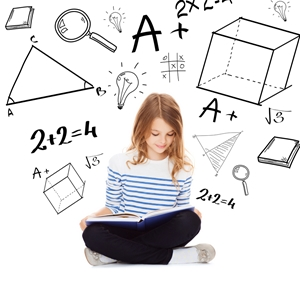# Math modeling connects the classroom to the real world

MONDAY, JANUARY 19, 2015 10:31 AMMany math teachers can relate to a common concern they hear in class: Students wonder whether formulas matter outside of school. Will they really use algebra in their career? Can geometry actually help them in the long run? These questions are fair, especially as students age and want to make sure their time is spent helping them prepare for college and careers. However, some still fail to realize that math actually does come in handy in life. The Common Core State Standards include a section that addresses the connection between school math and real life: math modeling.

Math in the real world
Students may struggle to look at their algebra homework and understand how these formulas act in future jobs. Math modeling is a practice in which users devise a model to help them solve problems they may encounter in life using math. For instance, students can create a model to measure how many desks will fit in a classroom. They'll have to use formulas for area, but the math is applicable to a situation with which they can identify.

Math modeling can go beyond simple computation to help students figure out a variety of numbers. Not only will they be able to calculate how many desks fit in a room, but how much other furniture, the best layout for desks and chalkboards, etc. Math modeling gives students a tool for discovering a variety of answers.

A challenging subject
The Common Core includes math modeling benchmarks starting in high school. Applying math skills to real world scenarios can be challenging, so it's reserved for more advanced students. However, younger children still use modeling to some degree. They might look at a picture of a box that has measurements written on it and have to calculate area.

Moreover, math modeling isn't set up like other topics in the Common Core. Rather than have a set of unique benchmarks, math modeling is intertwined with other topics. That way, teachers can use modeling while also teaching high schoolers algebra and geometry.

Creating a model
The Common Core lists steps that students must take when developing a math model. They include:

1. Breaking down variables in a problem and identify which are essential to solving it
2. Using statistical models to represent relationships between variables
3. Running calculations to draw conclusions
4. Interpreting the results
5. Comparing the numbers to the situation to see if they make sense, then adjusting model as needed
6. Sharing conclusions and the steps that got students to the answers

Building math models may help students see how math and the real world intersect.RELATED ARTICLES All About TerraNova 3 Testing Teaching gifted kids in normal classes Helping your kids continue learning this summer More..
 NEWS CATEGORIES NEWS ARCHIVE